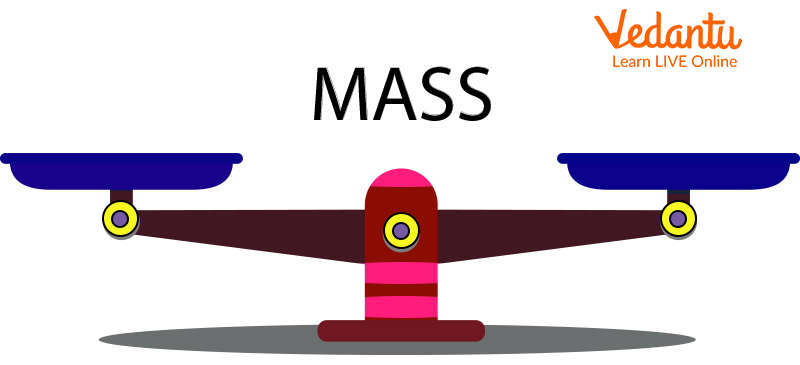Courses
Courses for Kids
Free study material
Free LIVE classes
More

# Definition of Mass in MathematicsLIVE
Join Vedantu’s FREE Mastercalss

## What is Mass?

In Mathematics, mass refers to the quantity of matter in an object. Mass is generally measured by how much something weighs. The more matter something has, the more it will weigh. For example, the mouse has more matter than an ant, hence, the mass of a mouse will be higher.

Remember, the size of something does not determine that the particular object has more matter. For example, although both golf balls and ping-pong balls are of the same size, the golf ball has more mass as it has more than a ping-pong ball. Also, the golf ball has more mass because it is solid and the ping-pong ball is hollow.Measuring Unit Mass

## Mass Definition In Maths

Mass can be best defined as the amount of matter present in any object or a person. Everything present around us has mass. For example, a chair, a table, a pen, a glass, etc. Remember even air has mass in it.

From the mass definition given above, we can say that an object's heaviness or lightness depends on its mass.

## Mass Example

The gold bar given below looks quite small but has a mass of 1 kilogram or 2.2 pounds.1 Kg Gold Bar

## How is Mass Measured?

Mass is measured in Kilograms, grams, and tonnes (Metric Units) or Ounces and Pounds (US Customary Units).

## What is the Standard Unit of Mass?

The standard unit of mass is kilogram. Kilogram is abbreviated as Kg. The unit kilogram is used to measure the mass of heavy objects such as bags of grains, stones, vegetables, etc.

On the other hand, the smaller unit of mass is grams. Grams is abbreviated as G. It is used to measure the mass of lighter objects such as a quarter teaspoon of sugar, a small paperclip, one US bill, etc.

Remember:

1 Kg = 1000 g or 1000 g = 1 Kg

Are Both Mass and Weight the Same?

No, both mass and weight are not the same things. Mass is referred to as the quantity of matter an object has whereas weight is referred to as the Gravitational force with which the Earth attracts the masses towards its center.

You must have observed that people often use both mass and weight interchangeably because gravity is almost the same everywhere and we don’t often observe differences.

Note: The mass of an object is always the same everywhere. But weight increases or decreases due to the higher or lower gravity.

The mass of an object can never be zero whereas the weight of an object can be zero if no gravity acts upon it, such as in space.

## Conclusion

In short, mass is a measure of how much matter is there in an object. In the metric system, it is generally measured in grams, kilograms, and tonnes. Weight and Mass differ from each other. If you weigh something on another planet, its mass will be the same but its weight will be different. As weight depends on the gravity and gravity is different in every planet. Hence, when you are floating in space, you are weightless, but you still have a mass.

Last updated date: 22nd Sep 2023
Total views: 130.8k
Views today: 3.30k

## FAQs on Definition of Mass in Mathematics

1. How to measure the mass without a weighing scale?

When you don't have a weighing scale, you can measure weight by making a balance scale from a ruler and using coins from your pocket.

2. How to record mass?

One of the most common methods of measuring mass directly is to tare the scale and measure the mass. This is how people usually weigh themselves. The other common method of measuring mass is to place a sample into a container and measure the mass of the container along with the sample. The mass of the sample can be obtained by subtracting the mass of the container.